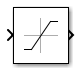# Saturation

Limit input signal to the upper and lower saturation values

•Libraries:
HDL Coder / Discontinuities

## Description

The Saturation block produces an output signal that is the value of the input signal bounded to the upper and lower saturation values. The upper and lower limits are specified by the parameters Upper limit and Lower limit.

InputOutput
Lower limit ≤ Input value ≤ Upper limitInput value
Input value < Lower limitLower limit
Input value > Upper limitUpper limit

## Ports

### Input

expand all

The input signal to the saturation algorithm.

Data Types: `double` | `single` | `int8` | `int16` | `int32` | `int64` | `uint8` | `uint16` | `uint32` | `uint64` | `fixed point`

### Output

expand all

Output signal that is the value of the input signal, upper saturation limit, or lower saturation limit.

Data Types: `double` | `single` | `int8` | `int16` | `int32` | `int64` | `uint8` | `uint16` | `uint32` | `uint64` | `fixed point`

## Parameters

expand all

### Main

Specify the upper bound on the input signal. If the input signal is greater than this boundary, then the output signal is set to this saturation value. The Upper limit parameter is converted to the output data type using round-to-nearest and saturation. Upper limit must be greater than the Output minimum parameter and less than the Output maximum parameter.

#### Programmatic Use

 Block Parameter: `UpperLimit` Type: character vector Value: real scalar or vector Default: `'0.5'`

Specify the lower bound on the input signal. If the input signal is less than this boundary, then the output signal is set to this saturation value. The Lower limit parameter is converted to the output data type using round-to-nearest and saturation. Lower limit must be greater than the Output minimum parameter and less than the Output maximum parameter.

#### Programmatic Use

 Block Parameter: `LowerLimit` Type: character vector Value: real scalar or vector Default: `'-0.5'`

Select this check box to cause the commands to treat the gain as 1. The linearization commands in Simulink® software treat this block as a gain in state space. Clear the box to have the commands treat the gain as 0.

#### Programmatic Use

 Block Parameter: `LinearizeAsGain` Type: character vector Values: `'off'` | `'on'` Default: `'on'`

#### Programmatic Use

 Block Parameter: `ZeroCross` Type: character vector | string Values: `'off'` | `'on'` Default: `'on'`

Specify the time interval between samples. To inherit the sample time, set this parameter to `-1`. For more information, see Specify Sample Time.

#### Dependencies

This parameter is visible only if you set it to a value other than `-1`. To learn more, see Blocks for Which Sample Time Is Not Recommended.

#### Programmatic Use

 Block Parameter: `SampleTime` Type: string scalar or character vector Default: `"-1"`

### Signal Attributes

The Data Type Assistant helps you set data attributes. To use the Data Type Assistant, click. For more information, see Specify Data Types Using Data Type Assistant.

Lower value of the output range that Simulink checks.

Simulink uses the minimum to perform:

Note

Output minimum does not saturate or clip the actual output signal. Use the Saturation block instead.

#### Programmatic Use

 Block Parameter: `OutMin` Type: character vector Values: `'[ ]'`| scalar Default: `'[ ]'`

Upper value of the output range that Simulink checks.

Simulink uses the maximum value to perform:

Note

Output maximum does not saturate or clip the actual output signal. Use the Saturation block instead.

#### Programmatic Use

 Block Parameter: `OutMax` Type: character vector Values: `'[ ]'`| scalar Default: `'[ ]'`

Choose the data type for the output. The type can be inherited, specified directly, or expressed as a data type object such as `Simulink.NumericType`. For more information, see Control Data Types of Signals.

#### Programmatic Use

 Block Parameter: `OutDataTypeStr` Type: character vector Values: ```'Inherit: Same as input'```, ```'Inherit: Inherit via back propagation'```, `'single'`, `'int8'`, `'uint8'`, `int16`, `'uint16'`, `'int32'`, `'uint32'`, `'int64'`, `'uint64'`, `fixdt(1,16,0)`, `fixdt(1,16,2^0,0)`, `fixdt(1,16,2^0,0)`. ```''``` Default: ```'Inherit: Same as input'```

Select this parameter to prevent the fixed-point tools from overriding the Output data type you specify on the block. For more information, see Use Lock Output Data Type Setting (Fixed-Point Designer).

#### Programmatic Use

 Block Parameter: `LockScale` Type: character vector Values: `'off' | 'on'` Default: `'off'`

Choose one of these rounding modes.

`Ceiling`

Rounds both positive and negative numbers toward positive infinity. Equivalent to the MATLAB® `ceil` function.

`Convergent`

Rounds number to the nearest representable value. If a tie occurs, rounds to the nearest even integer. Equivalent to the Fixed-Point Designer™ `convergent` function.

`Floor`

Rounds both positive and negative numbers toward negative infinity. Equivalent to the MATLAB `floor` function.

`Nearest`

Rounds number to the nearest representable value. If a tie occurs, rounds toward positive infinity. Equivalent to the Fixed-Point Designer `nearest` function.

`Round`

Rounds number to the nearest representable value. If a tie occurs, rounds positive numbers toward positive infinity and rounds negative numbers toward negative infinity. Equivalent to the Fixed-Point Designer `round` function.

`Simplest`

Automatically chooses between round toward floor and round toward zero to generate rounding code that is as efficient as possible.

`Zero`

Rounds number toward zero. Equivalent to the MATLAB `fix` function.

#### Programmatic Use

 Block Parameter: `RndMeth` Type: character vector Values: `'Ceiling'` | `'Convergent'` | `'Floor'` | `'Nearest'` | `'Round'` | `'Simplest'` | `'Zero'` Default: `'Floor'`

## Block Characteristics

 Data Types `double` | `fixed point` | `integer` | `single` Direct Feedthrough `yes` Multidimensional Signals `no` Variable-Size Signals `no` Zero-Crossing Detection `yes`

## Version History

Introduced before R2006a